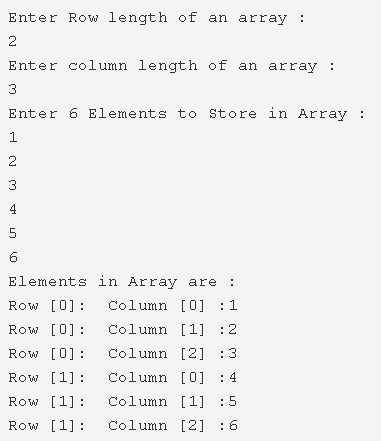# Two Dimensional Array In Java – JavaTutoring

Two Dimensional Array in Java Programming – In this article, we will explain all the various methods used to explain the two-dimensional array in Java programming with sample program & Suitable examples.

All the methods will be explained with sample programs and suitable examples. The compiler has also been added so that you understand the whole thing clearly.

The means used in this piece are as follows:

• Using Standard Method
• Using For Loop
• Using Scanner
• Using String

An array, as we all know, is a collection of multiple elements of the same data type. Arrays are normally used to store information of one particular type of variable.

A two-dimensional entity, in general, is something that has two specific parameters. Those two parameters are usually length and breadth since it is a physical quantity.

Similarly, a two-dimensional array is an array which technically has one row of elements, however, each row has a bunch of elements defined by itself.

Basically, you need to define both the rows and columns and then go ahead with declaring the elements in the respective locations or indexes.As you can see in the example given above, firstly, you need to specify the number of rows that you are assigning with the array.

• In this case, it is 2.
• Next, you need to mention the number of columns that you want to assign with the array.
• Here, it is 3.
• Thus, there will be a total of 6 elements that can go into a 2×3 Matrix.

The elements entered as per the example are as follows:

1

2

3

4

5

6

Hence, the elements are arranged accordingly and you will get your two-dimensional array.

## Two Dimensional – Using Standard Method

Output:

### Using For Loop

1. In two dimensional array represent as rows and columns.

2) To print the two-dimensional array, for loop iterates from o to i<3 for loop iterates from j=0 to j<2 print the element which is at the index a[i][j].

Output:

#### Using Scanner

1. Read the row length, column length of an array using sc.nextInt() method of Scanner class.

2) Declare the array with the dimension row, column.

3) for i=0 to i<row for j=0 to j<column sc.nextInt() reads the entered number and insert the element at a[i][j].

Output:

##### Two Dimensional – Using String
1. To print the elements of two-dimensional string array for i=0 to i<3 for j=0 to j<2 prints the string element which is at the index str[i][j].

2) Here i indicates row number and j indicates column number

Output:

x

## Plus Star Pattern Java Program | Patterns

Java program to print plus star pattern program – We have written the below print/draw ...